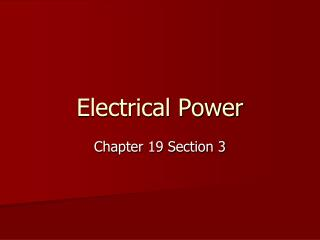DownloadDownload PresentationElectrical Power

# Electrical Power

Télécharger la présentation## Electrical Power

- - - - - - - - - - - - - - - - - - - - - - - - - - - E N D - - - - - - - - - - - - - - - - - - - - - - - - - - -
##### Presentation Transcript

1. Electrical Power Chapter 19 Section 3

2. Energy to Power • Electrical Power is the rate of conversion of electrical energy. • To push charge through a conductor, work must be done. • That work is a form of energy (Electrical Potential Energy) and when used over a certain time, power is created.

3. Electrical Power Equation • P = Power • I = Current • V = Voltage • R = Resistance

4. Electrical Companies • Electrical Companies measure energy consumed in Kilowatt-hours. • 1kWh = 3.6x106 J • The cost of a kWh can be between \$0.05 to \$0.20 • The average kWh in USA for 2011 is \$0.1099

5. High Voltage and Energy Transmission • Electrical Energy is transferred at high potential differences to minimize energy lost. • P = IV • P = I²R • By decreasing the current and increasing voltage in power lines it reduces the resistance of charge flowing through the power lines.